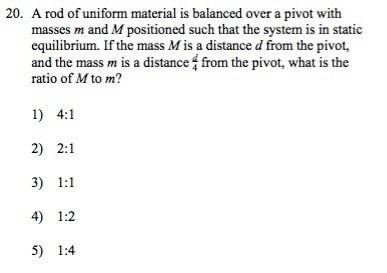# Question on torques. quick one.

• RoboNerd

## Homework Statement## Homework Equations

ok, I thought that the torque from the mass 'm' would be equal to torque from mass 'M'

## The Attempt at a Solution

I wrote down:

m * g * (d/4) = M * g * d

(m/4) = M

m = 4M

Thus, the ratio is 4M : 1 m, which gives answer 1.

However, the solutions say that the answer is 5.

Could anyone please weigh in, and thanks in advance for the help!

Thus, the ratio is 4M : 1 m
No, you have shown 4M=1m. Think of M and m as numbers there. What is M/m?

ahh 1/4. Right, thanks!

I have another method,
For the system to be in equilibrium the centre of mass should lie exactly on the pivot.

Assume the pivot to be at (0,0)
assume M to be at (d,0)
assume m to be at (-d/4 ,0)
Applying the formula of center of mass
x c.o.m. = (m(-d/4) +Md)/(m+M) = 0

Md = md/4
M:m=1:4

•CWatters and RoboNerd
That sir is an awesome and time-saving method! Thanks a lot! Everyone thanks!